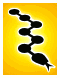# (Re-)Introduction to C for Pythonistas

Experienced / Tutorial
March 10th 9 a.m. – 12:20 p.m.
While Python is great for many things, C is still the lingua franca of the programming world. Whether you need to rewrite that critical loop or you want to get involved in CPython development, knowing the basics of C is an important skill for even the most hardened of Python coders. Over the course of this tutorial we will go over the basics of C and where Python programmers may get tripped up.

### Abstract

• Introduction (10 minutes)
• Environment setup (10 minutes)
• gcc basics
• Check for make
• Types (10 minutes)
• int, char, float
• unsigned keyword
• pointers
• void*
• arrays
• strings
• char*
• char[]
• structs
• unions
• enums
• Writing a function (10 minutes)
• Function signatures
• void
• Semicolon, semicolon, semicolon
• Declare variables at the top
• return()
• ints all the way down
• int main(int argc, char **argv)
• Exercise 1 (10 minutes)
• Quick overview of printf
• gcc -o
• Extend to ./ex1 name --> "Hello name"
• Blocks (10 minutes)
• if, while (same as Python, just mention for syntax)
• for
• do/while
• "simple" blocks
• Exercise 2 (10 minutes)
• ./ex2 hello --> "Hello world"
• ./ex2 list a b c --> "a\nb\nc\n"
• Use both if and for
• Confirm understanding of C-style for loops
• Preprocessor (5 minutes)
• #include
• "" vs. <>
• <stdio> vs. <stdio.h>
• #define
• #ifdef, #if
• #pragma once
• Headers (5 minute)
• How to declare a function
• Forward definitions
• Enums
• Exercise 3 (10 minutes)
• Must use multiple files
• ./ex3 10 --> "2 3 5 7"
• ./ex3 5 13 --> "5 7 11"
• Break (15 minutes)
• The compiler process (10 minutes)
• Preprocess
• gcc -D
• gcc -I
• Compile
• Assemble
• gcc -c
• gcc -l
• gcc -L
• make basics (15 minutes)
• Basic targets
• Dependencies
• Macros
• Suffix targets
• Exercise 4 (10 minutes)
• Create a makefile for ex3
• Useful functions (20 minutes)
• printf()
• %s, %i, %d, %f
• string.h
• strlen()
• strcpy() vs. strncpy()
• strncmp()
• malloc()/free()
• sizeof()
• calloc()
• realloc()
• memcpy(), memmove()
• stdio.h
• fopen()
• FILE*
• fwrite()
• fgets()
• fclose()
• snprintf()
• fprintf()
• stdint.h
• int32_t
• uint64_t
• math.h
• import math
• M_PI, M_E
• stdlib.h
• atoi(), atof(), strtol()
• random()
• exit()
• system()
• qsort()
• Comparator functions
• abs() (not in math.h)
• NULL
• size_t
• Runtimes (5 minutes)
• GLib
• Python
• C extensions, give some links
• C++
• STL
• Boost
• QtCore
• wxBase